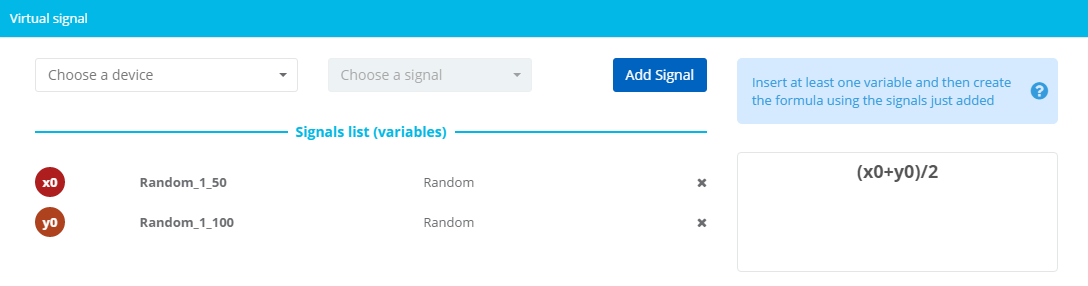# Virtual signals

A virtual signal allows the insertion of a formula to apply to other signals accessible to the user, to which variables are associated.For the syntax to use in the formula, refer to the apposite article.

Virtual signals are cached if they use more than a variable. Cache allows to calculate additional info, like the partial sum and average per value. If the virtual signal is not cached a notification is shown in the header of the section.

It is possible to execute advanced elaborations with particular formulas:

• Percentile, kf(percentile(x0,90),12) where 90 indicates the percentile (settable from 0 to 100) and 12 can be substituted with f to calculate the formula on the visualized periods;
• Arithmetic mean, kf(ma(x0),12) where 12 is the number of periods, settable to f to calculate the formula on the visualized periods resulting in a straight line;
• Weighted arithmetic mean, kf(wma(x0),12);
• Exponential mean, kf(ema(x0),12);
• Standard deviation, kf(ssd(x0),12);

### Using dates in formulas

With the proper syntax it is possible to evaluate dates in formulas.

eval(if(moment.tz(input.date, "America/New_York").hours()==14) result=18; else result = input.x0/2;)

The condition has to be included in eval(). To refer the date, the syntax is moment.tz(input.date, "TIMEZONE") where TIMEZONE, in TZ format (Wikipedia list), is the timezone to use in hour evaluation. It is possible to access the hour components with methods hours(), minutes(), and so on (Moment.js).

In the example above the input hour is evaluated with the "America/New_York" timezone, if hour is 14 then the result is setted to 18, else the signal value is  halved.